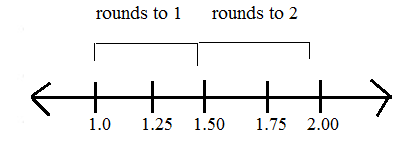# Rounding with Decimals

When we work with numbers, there are times, when the exact value is not as helpful as an estimate or a rounded amount. Instead, we round the value to a decimal that will be helpful. Let's take a look at a couple of different place values we can round to.

To the Nearest Whole

When we round to the nearest whole, this means that we are rounding the ones place. The final answer will not have any decimals in it.

123.45678

In this example, the 3 is in the ones place and the 4 is in the tenths place.

If we are rounding to the nearest whole, we look to the tenths place to tell us what to do. If the number is 5, or above, we give it a shove. In other words, we would round the ones place up. If the tenths place is 4 or below, we let it go. This means that we leave the ones place as it is and just let all of the other numbers after it go away.

This is an easy to remember little rhyme, but let's see why. When we have a decimal of 5 or above in the tenths place, we are actually closer to the next whole number on the number line.

When the decimal is 4 or below, we are closer to the original value on the number line.Here are some examples:

1.)239.2391

There is a 9 in the ones place and a 2 in the tenths place. The 2 tells us to leave the 9 alone. The rounded answer is 239.

2.)78.0341

There is an 8 in the ones place and a 0 in the tenths place. The 0 tells us to leave the 8 alone. So the final answer is 78.

3.)6712.832

There is a 2 in the ones place and an 8 in the tenths place. The 8 tells us to round the 2 up to a 3. So the final answer is 6713.

4.)8929.6512

There is a 9 in the ones place and a 6 in the tenths place. The 6 tells us to round the 9 up. Having a 9 in the ones place can make things tricky. Instead, think of the number as 29. When we round the 29 up we get 30. So we will replace the 29 before the decimal with a 30.

To the Nearest Tenth

We can use the same rules for rounding to different decimal places. Take a look at rounding to the nearest tenth.

1.)532.941

There is a 9 in the tenths place and a 4 in the hundredths place.
The 4 tells us to leave the 9 alone. 532.9 will be the final rounded answer.

2.)1294.77124

There is a 7 in the tenths place and a 7 in the hundredths place. The 7 tells us to round the other 7 up to an 8. That gives us 1294.8 as a final answer.

To the Nearest Hundredth

1.)9203.7234

There is a 2 in the hundredths place and a 3 in the thousandths. The 3 tells us to leave the 2 alone. Now we have 9203.7 as a final answer.

2.)78129.698124

There is a 9 in the hundredths place and an 8 in the thousandths place. The 8 tells us to round the 9 up. Instead of thinking of rounding just the 9, we can think about rounding up the 69 to a 70. So the final answer would be 78129.70 after rounding.

Let's Review:

No matter what place value you are asked to round to, you can use these same steps to get your final answer. If the number directly after the place value you need to round to is 5 or above, you round up. If the number is 4 or below, you leave the number alone.